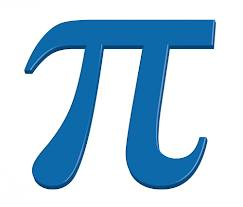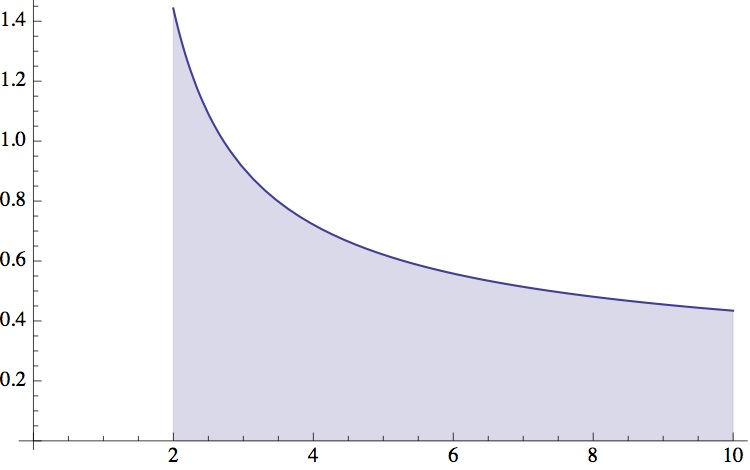# Think mathematics is (just) a language? Think again.

Posted by: Gary Ernest Davis on: March 25, 2013If you think mathematics is just a language, seek ponder this:$frac{pi}{4} = 1-frac{1}{3}+frac{1}{5}-frac{1}{7}+frac{1}{9}-frac{1}{11} +ldots = sumlimits_{n=1}^infty (-1)^{n-1}frac{1} {2n-1}$

and think again.

Courtesy of Chris Budd & +plus magazine

And if, see perchance, Â that is not convincing enough,Â try this: for a positive real number$x$ let$pi (x)$ denote the number of primes less than or equal to$x$, and let$extrm{Li}(x)=int_2^xfrac{1}{log (t)} dt$ be the area, from 2 to$x$,Â underÂ the graph of$frac{1}{log (t)}$:Area under curve$frac{1}{log (t)}$Â fromÂ t=2 to t=x (here, x=10)

Then:$limlimits_{x o infty}frac{pi (x)}{ extrm{Li}(x)} =1$

The rate at whichÂ$frac{pi (x)}{ extrm{Li}(x)}$Â approachesÂ 1 is aÂ majorÂ area ofÂ mathematicalÂ research, which is not advanced one whit by the view thatÂ mathematicsÂ is aÂ language.